Home > CCA2 > Chapter 8 > Lesson 8.1.3 > Problem8-57

8-57.
1. The x-intercepts of a quadratic polynomial are given below. Find a possible quadratic equation in standard form. Homework Help ✎

1. x =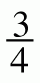, x = −2

2. x = −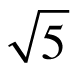, x =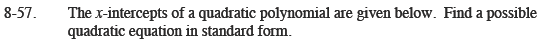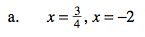Work backwards: Use the Zero Product Property to write an equation in factored form,
then multipy to write the equation in standard form.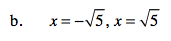Work backwards as was suggested in part (a).